Courses
Courses for Kids
Free study material
Free LIVE classes
More

# All About Graphs of Sine and Cosine Functions - JEE Important TopicLIVE
Join Vedantu’s FREE Mastercalss

## Introduction to Graphs of Sine and Cosine Functions

Before we go into the graphs of sine and cosine functions, we must first understand what these terms mean. Sine represents the ratio of the perpendicular and the hypotenuse, while the cosine function gives the ratio of the base and the hypotenuse. That being said, the sin and cosine functions are periodic functions that repeat themselves after a specific interval.

A periodic function is defined as the function for which a specific horizontal shift, P>0, results in a function which is identical and equal to the original function. This means that the same function repeats itself after a specific interval.

The mathematical expression is given as f(x+P) = f( x ) . This holds true for all possible values of x. The sine and cosine graphs are basically periodic functions where the period of the cosine function is the same as that of the sine function, which is equal to 2π.

## Graphs Related to Sine and Cosine Functions

### Plotting The Graph of Y = Sin (x):

The easiest way to plot the graph of y = Sin (x) is by drawing the table with the standard values of the sine function. The following table gives us the known values of the sine functions. Plotting the points of the values on the graph we get the  following curve :

 x 0 $\dfrac{\pi}{6}$ $\dfrac{\pi}{4}$ $\dfrac{\pi}{3}$ $\dfrac{\pi}{2}$ $\dfrac{2\pi}{3}$ $\dfrac{3\pi}{4}$ y 0 $\dfrac{1}{2}$ $\dfrac{1}{\sqrt{2}}$ $\dfrac{\sqrt{3}}{2}$ 1 $\dfrac{\sqrt{3}}{2}$ $\dfrac{1}{\sqrt{2}}$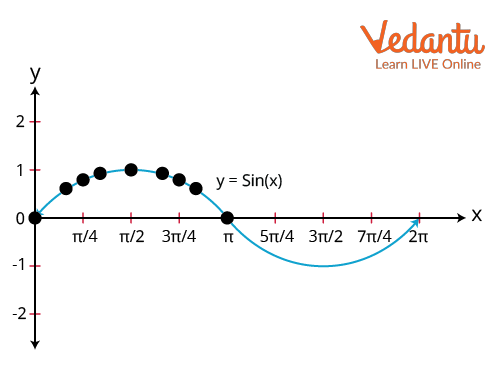Graph of the sine function

If you follow the graph closely you will see that the curve starts to repeat itself at

$x = 2\pi$. As mentioned before, trigonometric functions like sine and cosine expressions are periodic functions and the graph further emphasizes this point. This is because the angles that have a difference of $360^{\circ}$ between them have the same sine value.

Another method that can be used to draw the sine graph is the unit circle technique, a geometrical approach where a circle of radius 1 unit is considered that lies in the x-y plane. The points on the circle fulfill the criterion x2+y2=1. Any point lying on the circle has coordinates (x, y) where $x=\sin{\phi}$ and $y=\cos{\phi}$ (Here the angle $\phi$ is the angle subtended by the line from the origin to the x-axis). Thus, from the x coordinates we get the corresponding y coordinates of the unit circle, ultimately giving us the points to draw the sine graph.

Thus the final sine curve looks like a wave which is as follows:

 x 0 $\dfrac{\pi}{6}$ $\dfrac{\pi}{4}$ $\dfrac{\pi}{3}$ $\dfrac{\pi}{2}$ $\dfrac{2\pi}{3}$ $\dfrac{3\pi}{4}$ y 0 $\dfrac{1}{2}$ $\dfrac{1}{\sqrt{2}}$ $\dfrac{\sqrt{3}}{2}$ 1 $\dfrac{\sqrt{3}}{2}$ $\dfrac{1}{\sqrt{2}}$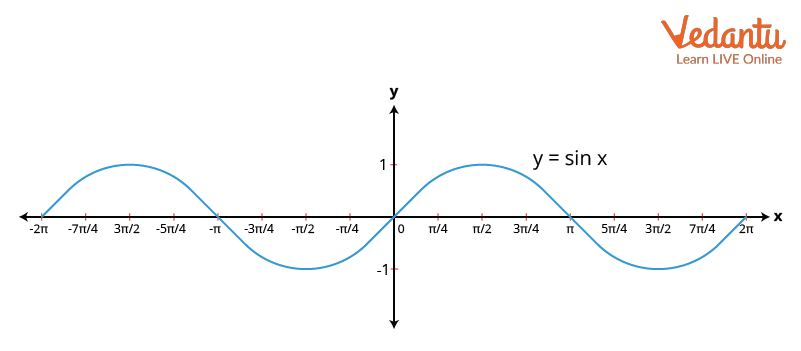Graph of sine function

### Plotting The Graph of y = cos x:

Just like the sin function, the graph of y = cos x can be drawn by plotting the points . The following table gives us some of the standard values of the cosine function and their respective y values. By plotting the points on the graph, we get the following cosine curve:

 y 0 $\dfrac{\pi}{6}$ $\dfrac{\pi}{4}$ $\dfrac{\pi}{3}$ $\dfrac{\pi}{2}$ $\dfrac{2\pi}{3}$ cos(x) 1 $\dfrac{\sqrt{3}}{2}$ $\dfrac{1}{\sqrt{2}}$ $\dfrac{1}{2}$ 0 $-\dfrac{1}{2}$Graph of Cos Function

## Period of Sinusoidal Functions

Before we go further into the topic, we must first be aware of some of the fundamental characteristics of the sinusoidal function. The following key points will give you a better understanding of what exactly is a sinusoidal function.

• Any function that has the same shape as that of either the sine or the cosine curve is termed a sinusoidal function.

• In both the graphs, the pattern of repetitions starts after a period of $2\pi$, which means that both the sine and cosine functions have a period of $2\pi$.

Therefore, $\sin{(2\pi+\theta)}=\sin{\theta}$ and $\cos{(2\pi+\theta)}=\cos{\theta}$.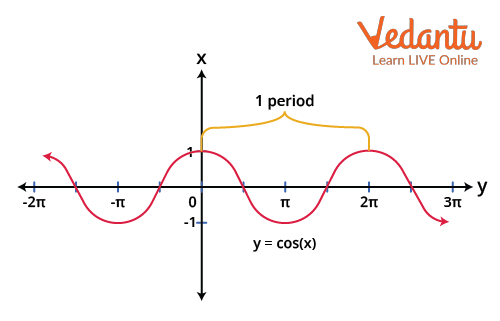Graph of y = sin ( x) showing the period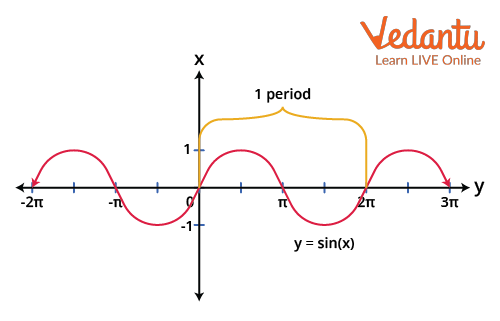Graph of y = cos ( x) showing the period

Let us consider another example in this context.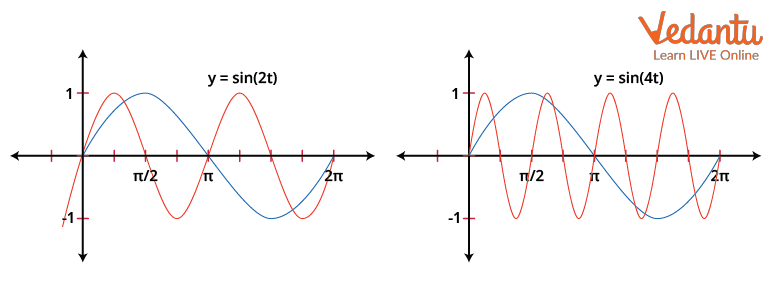Graph of y = sin(2t) and y = sin (4t)

From the above graphs, we can figure out that the graph of y = sin( 2t ) completes one cycle from 0 to $\pi$ and therefore exhibits a period of $P=\pi=\dfrac{2\pi}{2}$.

The graph of y = sin ( 4t ), similarly repeats itself over a period of $\left[0,\dfrac{\pi}{2}\right]$ and thus has a period of $\dfrac{\pi}{2}=\dfrac{2\pi}{4}$.

## How To Find Amplitude of a Graph?

The amplitude of any function is the magnitude of the wave. On a graph sheet, the value of the y coordinate or the ‘height’ of the curve is also a representation of the amplitude. The  amplitude of a sinusoidal function y = A sin (Bx – C) + D is the absolute value of the parameter A. Similarly, for the sinusoidal periodic function y = A cos (Bx – C) + D, the amplitude is the mod value or the absolute value of A.

The easiest way to calculate the amplitude from a graph of any curve is simply by figuring out the maximum and the minimum points on the graph,  that is the crest and the trough. The amplitude can also be defined as the difference in the height between the top-most point and the horizontal axis and the bottom-most point of the curve to the horizontal axis and is given by the formula:

|A| = amplitude  = $\dfrac{1}{2}$ | maximum – minimum |.

The horizontal line that divides the curve into two exact halves is called the midline. For a uniform periodic function, where the distance of the crest and trough are equal from the midline, the amplitude is given as the height measured from the midline to either the crest or the trough.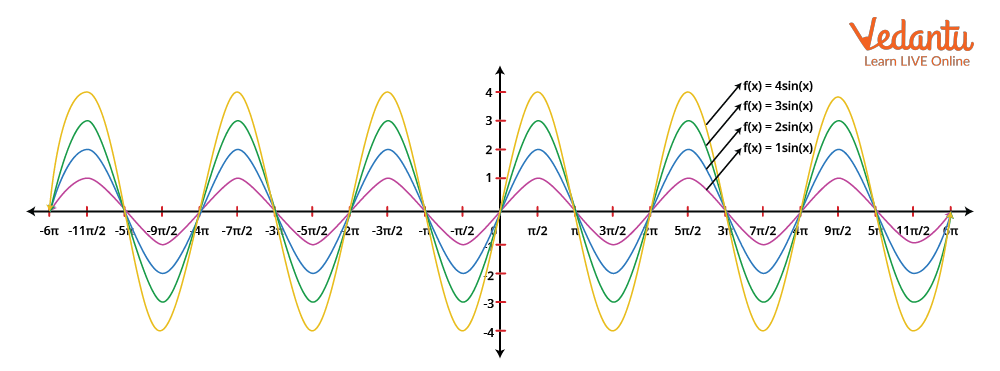Graph showing amplitudes of different sine functions

## Conclusion

The sine and cosine functions are periodic functions. These functions get repeated after a particular interval. The period of both the sine and the cosine curves is 2π. The period of sinusoidal functions is the interval after which the function comes back to the original function. The graphs can be drawn either by using the points whose sine values are known or by implementing the unit circle method. The amplitude of any function is calculated by measuring the distance between the midline which is the line dividing the graph into two equal halves and either the crest or the trough of the curve.

Last updated date: 20th Sep 2023
Total views: 132.9k
Views today: 2.32k

## FAQs on All About Graphs of Sine and Cosine Functions - JEE Important Topic

1. When is the negative cosine graph positive?

The negative cosine graph is positive in all the three quadrants, i.e., quadrants 1, 2 and 4. The third quadrant is the only portion where the negative cosine graph holds negative. The values for cos in the fourth quadrant are all positive. This can be summed up in the following way: Cos is positive in the fourth quadrant, All is positive in the first, Sin is positive in the second, and Tan is positive in the third.

2. What significance does the current issue have in terms of board exams? How much weight does it have in the JEE exam?

In the board exam, the current topic, Graphs of Sine and Cosine Functions, has intermediate levels. Since the board test is subjective in nature, the theoretical and numerical sections of this text are critical from an exam standpoint. Some numerical information is also available from the score advantage section.

The weightage of the Graphs of Sine and Cosine Functions is moderate because it only comprises a small amount of mathematics. The Graphs of Sine and Cosine Functions  is a simple idea that is used in this topic. Every year 2 to 3 questions from Graphs of Sine and Cosine Functions are asked in the JEE exam.